Impact Factor 3.560 | CiteScore 3.1
More on impact ›

# Frontiers in Physics## ORIGINAL RESEARCH article

Front. Phys., 13 May 2021 | https://doi.org/10.3389/fphy.2021.636052

# Collective Dynamics in Quasi-One-Dimensional Hard Disk System

• 2Institute for Condensed Matter Physics, National Academy of Sciences of Ukraine, Lviv, Ukraine
• 3Institute of Applied Mathematics and Fundamental Sciences, Lviv Polytechnic National University, Lviv, Ukraine
• 4Institute of Physics, National Academy of Sciences of Ukraine, Kyiv, Ukraine

We present the results of molecular dynamic studies of collective dynamics in a system of hard disks confined to a narrow quasi-one-dimensional (quasi-1D) channel. The computer simulations have been performed for the specific channel width of 3/2 of disk diameter in which the disk arrangement at close packing resembles zigzag ordering characteristic of a vertically oriented two-dimensional (2D) triangular lattice. In such a quasi-1D system, which is intermediate between 1D and 2D arrays of hard disks, the transverse excitations obey very specific dispersion law typical of the usual optical transverse modes. This is in a sharp contrast both to the 1D case, where transverse excitations are not possible, and to the 2D case, where the regular shear waves with a propagation gap were observed. Other peculiarities of the dispersion of collective excitations as well as some results of disk structuring and thermodynamics of the quasi-1D hard disk system are presented and discussed for a range of hard disk densities typical for fluid and distorted crystal states.

## 1. Introduction

Hard spheres and hard disks are widely accepted as the first choice approximation to model a variety of soft condensed matter objects . In spite of being a rather simple representation of the substance, hard core-based model systems still are capable to recover a number of basic properties and effects related to the structure, thermodynamics (e.g., phase transitions), and dynamics of real systems. Recently, the interest has been revived in the properties of hard sphere fluid confined to a narrow channel of the width that does not exceed two hard core diameters. In such system, commonly referred to as quasi-one dimensional (quasi-1D) system , the hard-core particles cannot pass the nearest neighbors and their motion is restricted by the neighbors. Of a particular interest is the so-called single-file quasi-1D system with the width that does not exceed $\left(1+\sqrt{3}/2\right)$ of disk diameter  as then a disk cannot touch more than one neighbor from each side. This is a substantial simplification that allows one to make a contact with the exact Tonks solution for the purely 1D hard rod system . The reasons for the interest to this system are both basic and applied. The fundamental interest stems from the existence of the analytical transfer matrix approach for the isobaric partition function  and the exact canonical partition function of this system, which is recently derived in . At present, the theoretical research in this area has been mostly concerned with the efforts (i) to get an insight into the mechanism that governs transformation of the properties of hard core systems as their dimensionality approaches 2 and 3 [5, 11], and (ii) to consider these systems as glass formers, see [79, 1214] and review . The practical interest stems from the possibility to use such a simple model to capture properties of more complex systems, e.g., to explain diffusion in zeolite and carbon channels , microfluidic devices , in the technology of bio-integrated nanodevices  etc. by treating the finite length axis of the quasi-1D system as the pore width.

Confined many-particle systems are very versatile and complex, and computer simulations are a perfect and in the most cases only available tool to study their structure and dynamics. It has been widely applied to study the structure and single-particle dynamics in quasi-1D systems with various model smooth/continuous particle–particle and particle–wall interaction potentials. But even the quasi-1D systems partially involving hard core particles and the quasi-1D systems of pure hard core particles (that are the main subject of present study) can be so different in their physics that comparison of apparently similar effects in such systems often has no much physical sense. For instance, in a quasi-1D system of hard core particles suspended in a viscoelastic liquid solvent their effective interactions are mainly of a hydrodynamic nature and the role of confining walls is not so much in restricting particles' motion as in setting the boundary condition on the liquid solvent's flow. At the same time, in a quasi-1D system of pure hard core particles all interactions are of an entropic nature. Another example concerns a zigzag particle arrangement that very often is the object of fundamental interest for different quasi-1D systems. However, because of the difference in interaction potentials, the general idea of the zigzag geometry is often the only thing in common. For instance, a zigzag structure can occur both in quasi-1D system with extremely short-range interaction such as a hard core repulsion (e.g., see ) and in quasi-1D system with long-range interaction such as a screened electrostatic repulsion in dusty plasma (e.g., see review ). The difference between these two interactions is known to be fundamental even in a purely 1D geometry in which the phase transition exists only for a long-range particle–particle repulsion . At the same time, the computer simulations studies of dynamic properties of the quasi-1D systems that contain only hard core particles, i.e., the quasi-1D hard core systems, have been primarily performed in the context of glassy dynamics. They were mostly focused on the problems specific for the single particle dynamics such as disk hopping time between different quasi-equilibrium states and defects' dynamics [7, 9, 12], and time dependence of the particle displacement [9, 13]. The glassy behavior of hard disk fluid confined to a narrow hard wall channel was also studied theoretically in  by analyzing the transverse and longitudinal equilibrium static pair correlation functions by the transfer matrix approach .

Our recent computer simulations studies [22, 23] have addressed the collective time correlation functions in the bulk 2D and 3D hard core systems. What in the following is referred to as collective dynamics means correlations in the cooperative motion of a many-particle system. Such an approach to understanding the dynamical processes is general for studies of propagating waves (sound, shear, and heat ones) and relaxation processes (thermal relaxation, structural relaxation, stress relaxation, etc.). The corresponding collective dynamic variables are defined via fluctuations of the conserved quantities: number of particles, longitudinal and transverse components of total momentum, and energy. All these collective dynamic variables are known from the hydrodynamic approach and can be used for theoretical description of the long-wavelength processes. For the theoretical description of collective dynamics beyond the hydrodynamic regime, the set of collective variables is extended by the orthogonal ones (orthogonal to hydrodynamic variables), which represent the longitudinal and transverse components of stress tensor, energy current, etc. More precisely, computer simulations [22, 23] were used to find how collective dynamics of bulk hard spheres and bulk hard disks behaves on different spatial scales. Rather unexpectedly the short-wavelength shear waves were found in both cases from the well-defined peaks of the transverse current spectral functions. Although nature of shear waves in hard-core systems appears to be essentially the same as in simple fluids , i.e., they emerge as the short-wavelength excitations due to coupling of the transverse current and transverse component of stress tensor, the hard-core fluids are known for the absence of viscoelastic effects. Interestingly, while there are no short-wavelength shear waves in a 2D hard disk fluid at low particle densities, these were observed in the range of higher densities by showing certain particular features just before the freezing transition.

The present paper is devoted to a quasi-1D hard disk system. An essential difference between the methods to study collective dynamics in hard-core systems and those for models based on analytic/continuous two-body potentials stems from the absence of a local energy minima in the former case. Even in the case of solids composed of hard spheres or hard disks, the particles do not oscillate around the minimum of potential well, but are moving ballistically in the cage formed by the nearest neighbors. This difference gives rise to an interesting specific aspect of the collective dynamics in a hard core system, namely, an existence of strong correlations between the emergence of short-wavelength shear waves and the caging phenomenon [25, 26], which was noticed in the case of 2D hard disk system . As caging inevitably emerges in a hard disk system under quasi-1D confinement, it is quite natural to expect the existence of short-wavelength shear waves in this case as well. Then it is not clear how the collective modes behave when additional confinement is imposed, how the reflections from channel boundaries affect the longitudinal and transverse excitations originated from particle–particle collisions, how the single-particle ballistic motions sum up to form a collective oscillation modes. The case of transverse excitations is of particular interest since latter are not present in the 1D prototype of the quasi-1D hard disk system. All these issues remain unexplored. Therefore, our aim is to perform molecular dynamics simulations of a quasi-1D system of hard disks, calculate its static structural properties, and make a link with the collective dynamics of the system. The rest of the paper is organized as follows. In the next section, we present the information on molecular dynamics simulations; section 3 contains results of the static and dynamic properties of the studied systems. In the last section, we discuss our findings.

## 2. Modeling and Simulation Details

The quasi-1D system is modeled by placing N hard disks of diameter σ into elongated rectangular box formed by two walls (lines) of length LxL that are separated by a distance LyH = σ + h with h < σ such that disks cannot pass each other. The disk-disk two-body interaction is given by

where rij = |rjri| is the distance between disk centers. Additionally, both confining walls are impenetrable,

thereby forming the hard-wall channel (or pore) of the width H = σ + h and of the length L. The ends of the channel are open and the periodic boundary conditions in x-direction are employed.

Taking into account that width H = σ corresponds to 1D case, the range of channel widths σ < H < 2σ could be considered as a bridge between 1D and higher dimensions. Among continuous variety of the quasi-1D system width in this range σ < H < 2σ there are two, H/σ = 1.5 and $H/\sigma =1+\sqrt{3}/2$, when disk ordering at close packing is commensurate with 2D triangular lattice like milestones on the way from 1D to 2D. To illustrate, Figure 1 presents two triangular hard disk arrays that correspond to the most common horizontal (Figure 1A) and vertical (Figure 1B) orientations of 2D triangular lattice that differ by an angle of 30 degrees. The quasi-1D systems that correspond to H/σ = 1.5 and $H/\sigma =1+\sqrt{3}/2$ are shown by hollow disks that at close packing form two very distinct crystalline zigzags. The main distinction concerns a number of nearest neighbors interacting with each disk, i.e., two for H/σ = 1.5 and four in the case of $H/\sigma =1+\sqrt{3}/2$. The narrower quasi-1D systems is more close to the 1D system, while wider quasi-1D system is more close to the 2D system. In the present study, we are considering the narrower quasi-1D system with width fixed at H/σ = 1.5.

FIGURE 1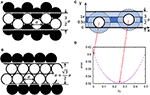Figure 1. Disk zigzag ordering in a vertically (A) and horizontally (B) oriented 2D triangular lattice at disk close packing. In the present study, we are employing the quasi-1D hard disk system of width H = σ + h with h/σ = 0.5 that at disk close packing resembles zigzag ordering shown in part (A). The driving force of such a zigzag ordering is entropy, which in the case of hard disk system confined by hard walls is uniquely determined by excluded volume, as it is illustrated in (C,D). In the case of 1D or 2D hard disk systems, excluded volume (the patterned area of width σ/2 around the disks and near the walls) depends on the distance between disks, i.e., on the disk density only. For the quasi-1D system hard disk, the distance between disks and wall enters the play. In part (D), we illustrate how area (in some relative units), accessible to the centers of other disks (the area of channel filled in blue) depends on the transverse position y0 = yih of the center of one individual disk. One can see that such an area increases when disk moves to the channel walls and is minimal for its position at the middle of channel.

The main body of simulations, in particular, those that concern the collective dynamics, were performed by using a collection of N = 200 hard disks at the fixed channel width H with h/σ = 0.5 and the varied length, L/σ = 400, 350, 300, 250, 220, 198, 190, and 180. Thus, eight quasi-1D hard disk systems that are characterized by different disk linear density,

were simulated. For convenience of comparison and discussion, particular values of the linear density l, that corresponds to each channel length L, are shown in Table 1 together with corresponding values of the disk number density ρ = 2/(HL) and packing fraction η = Nπσ2/(4HL), since the latter are often used in the literature on quasi-1D systems. The simulation runs with larger systems up to N = 2, 000 were performed as well and are properly indicated in the text. Throughout the paper, the disk hard core diameter σ is used as unit of length, while time is in units of (βmσ2)1/2. In simulations we used β = σ = m = 1, where β = 1/kT and m is the mass of a disk.

TABLE 1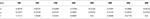Table 1. Density parameters (packing fraction η, number density ρ and linear density l) of the quasi-1D hard disk system with channel width H/σ = 1.5 and varied channel length L for the case of N = 200 disks.

Initially chosen N disks were located randomly inside the channel of the shortest length L, i.e., the highest density considered. Then initial disk configurations for lower densities were obtained by increasing the channel length L. To handle the collisions of hard disks with each other and with the channel hard walls, we employ the event driven molecular dynamics (MD) algorithm [27, 28]. According to this technique, the temperature is kept constant by scaling appropriately the magnitude of velocities of each hard disk such that kinetic energy of the system agrees with the equipartition theorem. The directions of the velocities of disk particles at the beginning of each run were chosen randomly. Before calculating the averages for static quantities, we ran MD simulations with assigned velocities, as described before, to equilibrate the system.

Collective dynamics was studied via calculation of the density-density, energy-energy, and longitudinal (L) and transverse (T) current–current time correlation functions. In order to get equally time-sampled positions of hard disks, i.e., the disk trajectories, the positions of hard disks between the collisional events were interpolated. Proceeding in this way, for the systems of N = 200, 400, and 1,000 hard disks, we recorded the trajectories and velocities along trajectory for each hard disk, totally having dumped 100, 000 configurations for the systems of N = 200 particles, but 40,000 configurations for the systems of N = 400 particles, and only 20,000 configurations for the systems of N = 1, 000 particles with the fixed reduced time interval of 0.01.

The following Fourier components were sampled for each configuration. The Fourier component of the particle density

of the longitudinal component of current density

and the transverse component of current density

as well as of the energy density

Here xi is the position of disk i along the channel, vx, i(t), vy, i(t) are the components of the ith disk velocity along the channel and perpendicular to the channel, respectively, while ${\epsilon }_{i}^{kin}$ is the kinetic energy of ith disk at time t. The wave vector k is defined along the channel x−axis, compatible with the periodicity of simulation box, as kkx = 2πm/Lx with m = 1, 2, 3, …. Note that, due to a non-zero channel width H, the positions and velocities of particles in quasi-1D systems have two components, along (x) and perpendicular (y) to the channel. However, we are using only wave vectors sampled along the channel. The reason is that transverse collective excitations in atomistic systems can propagate if at least two particles take part in the transverse collective motion, i.e., the wave number is below π/σ. In the case of a narrow channel of width H = 1.5σ the smallest wavenumber, ky = 2π/H, is too large.

Having the Fourier components of energy and particle densities, it is straightforward to calculate the Fourier components of the heat density 

Here fne(k) and fnn(k) ≡ S(k) are the static energy–density and density–density correlators, respectively, while S(k) is the static structure factor. The fluctuations of heat density permit calculations of wavenumber-dependent specific heat at constant volume Cv(k), which in the long-wavelength limit tends to its macroscopic value. Another important quantity that can be obtained from observed heat density dynamics is the Landau–Placzek–like ratio, which gives information on the share of contributions from relaxing and propagating processes to specific heat Cv [24, 29].

The above-defined time-dependent Fourier components of corresponding densities, equations 4 - 8, describe fluctuations of conserved quantities in monoatomic fluids and form the set of hydrodynamic variables of macroscopic collective dynamics. Having dynamic variables (4–8), we calculated the time-dependent density–density correlation function,

time dependent longitudinal and transverse current–current correlation functions,

and time dependent heat density autocorrelation function,

In what follows, the correlation functions (9–11) were used for numerical time-Fourier transformation in order to obtain the density–density dynamic structure factors S(k, ω), longitudinal/transverse current spectral functions, CL/T(k, ω) and the heat-density dynamic structure factor Shh(k, ω).

## 3. Results

The system of hard disks in a narrow hard wall channel is anisotropic . The anisotropy is expected due to the system setup L >> H, which results in two different pressure components, i.e., the longitudinal, PL = FL/H, and transverse, PT = FT/L. Here, FL and FT are the force per unit cross-section exerted along the channel length L, and the force on a segment of the horizontal wall of length L/() = 1/l, respectively. These forces are both of entropic origin and rather sensitive to be evaluated from computer simulations. Fortunately, these forces can be found from the analytical canonical partition function of a quasi-1D hard disk system reported in .

Figure 2 presents the dependencies PL(l) and PT(l), as well as FL(l) and FT(l) for three channel widths, H = σ + h, with h fixed at: (a) h/σ = 0.141, close to the 1D case; (b) h/σ = 0.5, which is far from both lower 1D and higher single-file limits; and (c) h/σ = 0.866, which is very close to the terminate width $h/\sigma =\sqrt{3}/2~0.866025$ of a single-file system. At low linear density, the transverse force FT is density independent, implying that the vertical disks' motion is ballistic, the vertical free path is maximum, i.e., H − σ, the disks bounce between channel walls and do not collide with other disks. As for the same low linear density l ≲ 0.8, the transverse force is lower for a wider channel, the frequency of disk bouncing between the horizontal walls has to be lower for wider channels as well.

FIGURE 2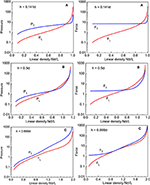Figure 2. Density dependence of the longitudinal (L) and transverse (T) pressures and forces in quasi-1D hard disk system of different channel width H/σ = 1.141 (A), 1.5 (B), and 1.866 (C) calculated using the analytical canonical partition function .

We see that, for low densities, the transverse pressure PT is higher than the one along the channel. This is because in that case PL is determined by a large disk separation along the channel, whereas PT is determined by a short range of transverse motion bounded from above by H − σ. As linear density increases toward l ≥ 1, disks start to mount one upon another, the vertical free paths decrease and transverse pressure and force both rapidly increase. For h/σ = 0.866, Figure 2C, this results in PT to be always higher than PL. However, for more narrow channels with h/σ = 0.141 and h/σ = 0.5, Figures 2A,B, before this happens, at certain value of linear density, which is different for each channel width H, the gaps between disks and walls in transverse direction and the gaps between neighbor disks along the channel become equal, and the pressures on the vertical and horizontal boundaries coincide.

The density distribution profiles, ny(y), in the transverse y−direction, obtained both from the analytical partition function  and from MD simulations for quasi-1D hard disk system of the width H/σ = 1.5, are shown in Figure 3. While linear density is low, l ≲ 0.8, the system is roughly homogeneous across the channel as the density profiles ny(y) are nearly constant and equal to the correspondent linear density l. In contrast, as linear density increases, l > 1, the disk distribution across the channel shows the tendency of increase in the regions close to the channel walls and decrease in the middle region of the channel. For the highest studied linear density, l = 1.111, the density profile ny(y) exhibits an almost δ−like shape in the close proximity of the channel walls and practically vanishes elsewhere.

FIGURE 3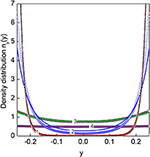Figure 3. Transverse density profile, ny(y), of the quasi-1D hard disk system with channel width H/σ = 1.5 and different disk linear density l: 1—1.111; 2—1.053; 3—0.909; 4—0.5. The symbols correspond to MD simulation data, while solid lines result from the analytical canonical partition function .

The longitudinal static structure factor, S(k), is calculated from MD simulations in a standard way as instantaneous-time density–density correlator,

In Figure 4, we show the changes in the first peak of S(k) with decrease of linear density l. Regarding the collective dynamics, one of the most important features is location Kmax of the main peak of S(k), since the value of k = Kmax has the meaning of a pseudo-Brillouin zone boundary in the considered quasi-1D hard disk fluid at a particular density. In this region of wavenumbers, de Gennes's slowing down of the density fluctuations takes place, which is ultimately reflected in the long tails of the density–density time correlation functions. One can see that for linear densities l > 1 the structure factor S(k) is typical for distorted crystals, with the main peak shaped as the sheared-out delta function. For linear densities l < 1, one observes typical fluid-like structure factor. In disordered systems where a structural transition takes place, one can find different slopes of the main peak of S(k) on both sides of the transition . In Figure 5, we show the main peak position Kmax of longitudinal static structure factor S(k) as a function of the linear size L of system that is proportional to the inverse 1/l of linear density. The location of the main peak is changing from Kmax ≈ 7 for the case of L/σ = 180 (the highest linear density l = 1.111) down to the value Kmax ≈ 4.5 for L/σ = 400 (the lowest considered linear density l = 0.5). Indeed, one can see that there is a kink in the behavior of the maxima positions, which occurs in the region of linear densities 0.8 < l < 1.01. According to Figure 2B, this range of linear densities corresponds in our system to the thermodynamic state, where the transverse pressure PT is lower than the longitudinal pressure PL. It worth to note that in a quasi-1D system with channel width H/σ = 1.866 the latter newer happens, i.e., always PT > PL (see Figure 2C), while there is range of linear densities, 0.93 ≲ l ≲ 1.01, where transverse force FT is slightly smaller than longitudinal force FL. This density range corresponds to packing fraction range from η = 0.35 to 0.45 (see Figure 10 of ), where the time dependence of the mean-square displacement starts to develop a power-law time dependence due to the slow diffusion of defects in the zigzag arrangement of disks, suggested in  as a mechanism for the α relaxation.

FIGURE 4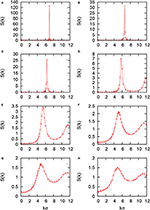Figure 4. Static structure factor, S(k) of the quasi-1D hard disk system with channel width H/σ = 1.5 calculated according to the definition equation 12 by using MD-generated 100,000 configurations of N = 200 disks. The sequence of panels (A–H) corresponds the linear density decreasing from l = 1.111 (A) to 0.5 (H) according to Table 1.

FIGURE 5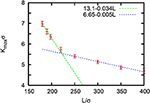Figure 5. Dependence of the main peak position, Kmax, of the static structure factor S(k) of quasi-1D hard disk systems with the channel width H/σ = 1.5 on the channel length L that is proportional to inverse 1/l of linear density.

The single-particle dynamics can be studied by means of the velocity autocorrelation functions

The velocity autocorrelation functions were already obtained by means of molecular dynamics simulations for the bulk 3D hard sphere  and 2D hard disk  fluids. In both cases, the authors found that there exists the packing fraction value (η = 0.45 for 3D case and η = 0.65 for 2D hard disks) above which the velocity autocorrelation function at short times develops a negative minimum signaling of a nascent caging. In our case, an anisotropic quasi-1D hard disk system shows essentially different behavior for the directions along and across the channel. In Figure 6, we show the velocity autocorrelation function ψ(t), its xx- and yy-components, and how they depend on the disk density. The xx-component, ψxx(t), in the quasi-1D hard disk system behaves in a way very similar to that for bulk 2D hard disk system . It has long-time tails for small linear densities l ≲ 0.667 and changes to a shallow negative minimum at the largest studied linear density l = 1.111 (packing fraction η = 0.582) due to collisions with the nearest neighbors.

FIGURE 6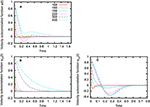Figure 6. Velocity autocorrelation function, ψ(t) (A) and its longitudinal (the xx direction along the channel) (B) and transverse (the yy direction across the channel) (C) components for quasi-1D hard disk system with channel width H/σ = 1.5 at different linear densities l = 1.111(L = 180), 1.053(190), 1.01(198), 0.909(220), 0.667(300), and 0.5(400).

The effect of reflections from the channel hard walls is well seen in the transverse component, ψyy(t), of the velocity autocorrelation function. It took us by surprise that the characteristic oscillation of ψyy(t) due to the wall reflections changed its shape, and especially so for the intermediate density l = 1.01. Toward shorter times, it became more shallow, transforming for the most dense system, l = 1.111, into a very high-frequency heavily damped oscillation. This effect is much better seen in the Fourier-spectrum ${\stackrel{~}{Z}}_{yy}\left(\omega \right)$ of the function ψyy(t) shown in Figure 7. The characteristic oscillation due to hard wall reflection is changed starting from l = 1.01 to shift toward higher frequencies with increasing oscillation damping. This means that at high densities the zigzag structuring of hard disks prevents their reflections from both channel walls. Instead under zigzag ordering hard disks are reflected from the single nearest wall and from two nearest zigzag neighbors.

FIGURE 7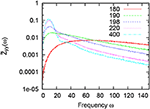Figure 7. Fourier-spectrum, ${\stackrel{~}{Z}}_{yy}\left(\omega \right)$, of the transverse velocity autocorrelation function, ψyy(t), shown in Figure 6C, at different linear densities l = 1.111(L = 180), 1.053(190), 1.01(198), 0.909(220), and 0.5(400).

The xx- and yy-components of the velocity autocorrelation function in a quasi-1D system were discussed so far only for the case of hard core particles suspended in a viscoelastic liquid solvent . Similar to our Figure 6B, the authors observed a negative minimum and a negative long time tail of the asymptotic form ~ −t−3/2 for the component parallel to the channel axis. They also concluded that both findings take place only for sticky boundary condition and does not occur if the solvent can slip over the walls. Our finding of a negative minimum of the velocity autocorrelation function in Figure 6B at the highest studied linear density l = 1.111 is of a completely different nature as our system consists solely of hard disks, which fly ballistically between hitting hard obstacles. We did try to estimate the exponentials for a negative long-time tail of ψxx(t). However, even in our case of saved 100,000 configurations with N = 200 particles, the noise in the tails was very strong, which did not allow us to reliably estimate the exponents. This shows that the lattice Boltzmann simulations  are more appropriate tool for this purpose than the Newtonian MD simulations.

Collective dynamics is usually studied via analysis of the time correlation functions, which store the entire information about collective excitations in the system and their coupling. So far, the collective dynamics has been well-understood on the macroscopic scales in bulk systems, but practically no information is available in the literature on collective excitations in confined low-dimensional systems consisting purely of hard-core particles. The time correlations in the latter case can be essentially different from that in the bulk systems. A simple example of this difference is seen in the transverse dynamics of considered quasi-1D hard disks system, which at the smallest wavenumber accessible in our simulations always shows damped oscillations due to reflection from the channel hard walls. In contrast, in bulk 3d hard-sphere and 2D hard-disk systems, where macroscopic hydrodynamics is valid, no transverse excitations exist at small wavenumbers accessible in simulations. It is seen in Figure 8 that the change of collective transverse current autocorrelation functions with disk density is practically the same as for the single-particle yy-velocity correlations. This makes it evidence that the leading contribution to the transverse current–current time correlation functions comes from the particle reflections from the channel hard walls.

FIGURE 8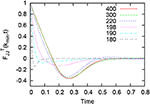Figure 8. Collective time correlation function of transverse momentum in quasi-1D hard disk system with channel width H/σ = 1.5 evaluated at the smallest wavenumbers kmin accessible in simulations, and for different linear densities l = 1.111(L = 180), 1.053(190), 1.01(198), 0.909(220), 0.667(300), and 0.5(400). The standard hydrodynamic shape of transverse current time correlation function in fluids is the single-exponential one.

The longitudinal current time correlation functions allow one to estimate the speed of sound for the smallest wavenumbers accessible in simulations. In Figure 9, we show the obtained dependence of the speed of sound on the channel length L that is proportional to inverse 1/l of linear density (see Table 1). As expected, the speed of sound shows a monotonic decrease with increasing channel length, i.e., with decreasing density.

FIGURE 9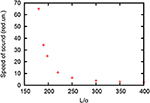Figure 9. Dependence of the speed of sound cs in the quasi-1D hard disk system with channel width H/σ = 1.5 on the channel length L that is proportional to inverse 1/l of the linear density.

Dispersion of the longitudinal and transverse excitations in the studied quasi-1D hard disk system were obtained from the peak positions of the longitudinal and transverse current spectral functions CL/T(k, ω) that are the time-Fourier transforms of the MD-derived longitudinal/transverse current–current time correlation functions ${F}_{JJ}^{L/T}\left(k,t\right)$. By employing well-established methodology , the latter were analyzed for their peak locations, which for different wave numbers k define the dispersion, ωL/T(k), of longitudinal and transverse excitations. Typical shapes of the spectral function CL/T(k, ω) are shown in Figure 10 for the system of N = 200 particles and linear density l = 1.01. As the shapes of the spectral functions are noisy, to locate their peak positions and maxima, we made use of the standard Bezier fit for noisy data. The simulations with larger numbers of particles allowed us to access smaller wavenumbers while the dispersion relations within the error bars remained the same.

FIGURE 10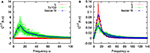Figure 10. Typical longitudinal (L) and transverse (T) current spectral functions, CL/T(k, ω), in the long-wavelength region at kmin = 2π/L (A) and for wave number k = 17kmin (B) for the system of N = 200 disks and linear density l = 1.01. In (A), the transverse spectral function was multiplied by a factor 100 for eye convenience. Blue line-connected asterisks show the Bezier fit applied for estimation of peak position of the noisy spectral functions.

Figure 11 show the dispersions ωL/T(k) of longitudinal and transverse excitations in the quasi-1D hard disk system. The parts of Figures 11A,B present data for the linear densities l < 1. At first glance in this case the dispersion ωL(k) of longitudinal excitations in quasi-1D hard disk system is very similar to that already observed for a 2D hard disk system . When linear density is low, l = 0.5, the dispersion ωL(k) only slightly deviates from being monotonic. But as soon as linear density increases, l = 0.909, it shows well-defined minimum around wavenumber values k ~ 6 associated with position of the main peak Kmax of longitudinal structure factor S(k) discussed in Figures 4, 5. The deviation from hydrodynamic dispersion law in the long-wavelength limit for both considered densities persists to be “negative.” The “negative” dispersion concerns the negative deviation of the dispersion curve ωL(k) at the boundary of hydrodynamic regime from the linear hydrodynamic dispersion law of acoustic modes in considered quasi1-D hard disk system. These effects are similar to those observed in 2D hard disk system with density increase . In contrast, the dispersion ωT(k) of transverse excitations is essentially different from those in the case of 2D hard disk system . Namely, for both linear densities l < 1 we are observing rather flat shape of the curve ωT(k) at reduced frequency ~10 with a tendency toward higher frequency values with increase of linear density. In 2D hard disk system, the transverse excitations are of acoustic nature. Moreover, the transverse excitations are absent at low densities and there was observed a long-wavelength propagation gap when they start to appear at higher densities. It is therefore quite natural to attribute the flat transverse mode in a quasi-1D hard disk system to disks' reflections from the channel hard walls. This issue is discussed in more details below.

FIGURE 11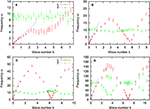Figure 11. Dispersions of longitudinal (L) and transverse (T) excitations, ωL(k) and ωT(k), in quasi-1D hard disk system with channel width H/σ = 1.5 at disk linear density l = 0.5 (A), 0.909 (B), 1.01 (C), and 1.111 (D). The dashed straight lines in the small-k region correspond to hydrodynamic dispersion law ω = csk with the corresponding speed of sound cs shown in Figure 9. The raw data for parts (C,D) were taken from our preceding paper .

Parts (c) and (d) of Figure 11 show similar data for the dispersions ωL/T(k) of longitudinal and transverse excitations but for the range of linear densities l > 1. As for the dispersions of longitudinal excitations, ωL(k), we see the tendencies already observed in Figures 11A,B under increase of linear density, i.e., the magnitudes of ωL(k) maxima are increasing while minima become deeper, reaching zero-frequency values at density ρ = 1.111 and being shifted toward larger wavenumber values k. The later again is consistent with the shift for the position Kmax of the first peak of the longitudinal structure factor S(k) in Figures 4, 5; the “negative” dispersion in long-wavelengths region is preserved as well. Such behavior of ωL(k) resembles one for the ordered solids and in  is interpreted as a consequence of the emergence a zigzag ordering in a squeezed quasi-1D system. The dispersion of transverse excitations in Figures 11C,D does not show notable changes too when the linear density was changing to l = 1.01. However, it does show dramatic changes at the highest considered linear density l = 1.111. Namely, (i) there is a sharp increase of frequency ωT up to ~70; (ii) the dispersion curve ωT(k) itself exhibits bubble-like shape by splitting on low- and high-frequency branches in the range of k-values that coincide with location of the maximum of dispersion ωL(k) that implies possibility of longitudinal-transverse excitation coupling on atomic scale in a squeezed almost zigzag ordered quasi-1D hard disk system.

The MD simulations allow one to study the fluctuations of heat density in the system and their effect on collective dynamics. We would like to remind that an adiabatic propagation of sound in fluids causes small deviations of the local temperature and instantaneous temperature gradients, which give rise to relaxation processes of the local temperature via thermal diffusivity. These relaxation process is directly connected with entropy fluctuations and is responsible for the central peak of dynamic structure factors S(k, ω). In Figure 12, we show dynamic structure factors S(k, ω) as well as heat-density dynamic factors Shh(k, ω) for three, the lowest wavenumbers in the quasi-1D hard disk system at the highest linear density l = 1.111. In both types of spectral functions, the side peaks are caused by longitudinal acoustic excitations, while the central peak for liquid state is caused by entropy fluctuations. In our case of the confined, almost zigzag structure at linear density l = 1.111 (channel length L/σ = 180 and width H/σ = 1.5), the central peaks of S(k, ω) and Shh(k, ω) give evidence of the same temperature (sometimes called entropy) relaxation processes typical for fluid state. We calculated Shh(k, ω) for lower densities too, and observed practically the same shape of Shh(k, ω) but with a larger smearing of the central and side peaks in comparison with the higher densities.

FIGURE 12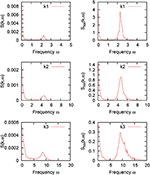Figure 12. Dynamic structure factor, S(k, ω), and heat-density dynamic factors, Shh(k, ω), of the quasi-1D hard disk system with the channel width H/σ = 1.5 derived from MD simulations at the highest considered linear density l = 1.111 for three the smallest wavenumbers k1 < k2 < k3.

## 4. Discussion

In dense, nearly solid-like states of a quasi-1D hard disk system, we observed a rapid increase of the transverse frequency ωT(k) in the long-wavelength region k ~ 0 from ωT ~ 12 for linear density l = 1.01 in Figure 11C to the frequency ωT ~ 70 for linear density l = 1.111 in Figure 11D. The shallow minimum in ωT(k) profile, observed for linear density l = 1.01 at wavenumbers k ~ Kmax/2, also deepens and becomes well-developed. Eventually, however, frequency ωT(k) at k ~ Kmax/2 splits into a high- and low-frequency branches at linear density l = 1.111 as shown in Figure 11D. The observed change in the dispersion ωT(k) of transverse excitations as the system changes from rarefied to dense can be explained by formation of the zigzag structure. The transverse low frequency mode, which is due to bouncing between the two hard walls, transforms into the high frequency transverse oscillations between one wall and the nearest neighbors in the zigzag structure. This is also supported by the behavior of the transverse component of the velocity autocorrelation function ψyy(t) in Figure 6C. Inspired by this intriguing behavior, one of us developed an analytical theory  of this system, which suggests that it is related to developing window-like defects in the zigzag disks' arrangement . The idea of defects of this kind (defect is a local less packing) has been introduced  and employed [79, 1214] to describe glassy dynamics in terms of caged and uncaged states in the disk arrangements in a quasi-1D hard disk system of the channel width H/σ = 1.866. In , such defects were associated with the maximum contact separation of two disks along the channel, which is equal to the disk diameter σ; in , their distribution was found analytically as a function of the linear density. As for the densities l > 1, the horizontal contact distance between disks and the actual horizontal distance between nearest neighbor disks are very close, this distribution can be well representative of the actual disks separations Δx, which is supported by the computer simulation data.

At dense/close packing, the disks form perfect zigzag. As confinement weakens, the tendency to the entropy increase results in an emergence of progressively larger number of window-like defects through which pairs of next neighbor disks uncage and exchange their vertical positions (Figure 13). This theoretical prediction of  has been confirmed from MD simulations data for the distribution of the actual distances Δx between next neighbor disks (Figure 14) and is in line with the earlier result . For high linear density, l = 1.111, these are distributed around Δx/σ ~ 0.89 close to the minimum possible contact distance along the pore, $\sqrt{3}/2\approx 0.87\sigma$. However at slightly lower linear density, l = 1.053, in addition to the widening of maximum at Δx/σ ~ 0.92 we see the appearance of a sharp subpeak at Δx/σ = 1. In contrast to the wide maximum drifting toward larger Δx, the subpeak always remains at Δx/σ = 1, although its shape is changing: it becomes more and more pronounced and finally exceeds the main peak, signaling approaching a fluid-like state. It points to the exclusive role that next neighbor disk center-to-center distance Δx = σ plays. Namely, creation of windows of the width of disk diameter σ (σ−windows) in the zigzag array, neither wider nor narrower, is the most effective way to gain entropy by uncaging two disks and making them extend their wondering to the whole channel width. The described mechanism of disk's caging/uncaging by their neighbors in quasi-1D hard disk system is fundamentally different to that in quasi-1D system with a long range screened electrostatic repulsion in the dusty plasma  where particles stay at finite distances and the zigzag can even transform into a straight line.

FIGURE 13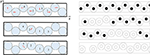Figure 13. Left: Disks' rearrangement in a pore that creates a window for two disks to exchange their vertical positions. Upper panel: disk in the pore at the average distance along the pore, which is below the disk diameter σ and disks cannot exchange their vertical positions. To let disk 1 go down, disks on the left and right of it get more dense. Mid panel: Disk 1 gets down through the window of size σ between disks 2 and 3. Now disk 2 may get up between disks 4 and 1. Lower panel: The exchange of the vertical positions of disks 1 and 2 is accomplished. Now disk 4 potentially can move down. Right: Representative snapshots of disk configurations taken from MD simulations of the quasi1-D hard disk system of the channel width H/σ = 1.5 to illustrate the schematics of disks' rearrangement. Shown are four the highest considered linear densities from the top to the bottom: l = 1.111, 1.053, 1.01, 0.909 that are discussed in Figure 14. The filled circles indicate disks that are caged and cannot exchange their vertical positions.

FIGURE 14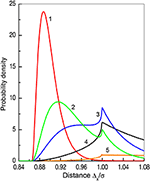Figure 14. Distribution of actual horizontal distances, Δx, between the nearest neighbor disks in quasi-1D hard disk system with channel width H/σ = 1.5 at disk linear densities l = 1.111(1), 1.053(2), 1.01(3), 0.909(4), and 0.5 (5) derived from MD simulations.

In a pure 1D hard disk system, the vertical motion is absent. In a densely packed quasi-1D hard disk system of the width H/σ = 1.5, it is also prevented by the full caging, but it eventually appears as the confinement weakens. As for sufficiently low density, the vertical disks' motion from one wall to another is possible, here one expects some contribution to the dispersion relation from the nearly ballistic transverse oscillation between the walls. As it comes from the maximum vertical path H − σ, this contribution ωT1(k) to the frequency ωT(k) at low density must be lowest possible. At high density, however, the σ−windows are rare and disks can bounce at most between one wall and the mid plane hence the lowest transverse frequency ωT2 for higher densities is expected to be roughly twice that for low density, ωT2 ~ 2ωT1. The frequency ωT2 is related to the maximal distance Δx at window nuclei, which require local compression and must result in high frequency longitudinal and transverse jitters. One can thus expect that the lowest transverse frequency ωT2 and the highest longitudinal and transverse frequencies appear near same wavenumbers k. In addition, the group velocity at this wavenumber range has to be zero as windows are not transferred by the waves. The dispersion of the longitudinal and transverse excitations in quasi-1D hard disk system obtained from MD simulations are in line with this picture (Figures 11C,D). For linear density l = 1.01, the peak at Δx = σ (Figure 14) indicates that σ−windows in the zigzag structure are well-developed, the disk order is of a short range, and frequency ωT1 can be identified with the practically k−independent transverse frequency ωT(Kmax/2) ~ 10 in Figure 11C. At the same time, for linear density l = 1.111, when there are almost no σ−windows (Figure 14), the transverse spectrum splits into the lowest, ωT2 ~ 20 ≈ 2ωT1, and the highest frequency, ωT ~ 100, at the wavenumbers k where longitudinal frequency is maximum (see Figure 11D). At wavenumbers k ~ Kmax/2, the curves ωT1(k) and ωT2(k) are plateaus, which indicate zero group velocities. The continuous longitudinal and transverse modes for linear density l = 1.111 are related to the short free path oscillation of mutually caged disks near the walls. Thus, the main properties of ωT(k), which are directly related to the vertical motion, are in line with the idea of the role of σ−windows in the zigzag arrangement.

The interpretation of computer simulation data in terms of the vertical disk motion presented above is also consistent with and well-illustrated by the theoretical dependence of the total transverse force FT(l) in Figure 2B. Indeed, as discussed above, for low linear density l, the transverse force FT is constant implying the vertical motion to be ballistic because the free path and free time are maximum. In contrast, for high linear density l, the transverse force FT sharply grows with l, the disks' arrangement is close to a dense zigzag, they cannot cross the middle line of the channel so that the free path and time at best are halves of their maximum values. Finally, this picture is obviously in line with the disk density distribution across the channel in Figure 3.

In conclusion, we would like to point out the novel and unexpected development, which stems from the above studies of the collective excitations. It is about a possible Kosterlitz-Thouless scenario in quasi-1D hard disk system . In 2D systems, melting proceeds via the Kosterlitz-Thouless scenario : a crystal develops defects, and their number is growing continuously from zero at zero temperature until the state becomes a liquid. The number of window-like defects in a zigzag arrangement behaves exactly in this way [7, 9, 10, 12]: it is zero only at dense packing and smoothly increases as density goes down. But in the Kosterlitz-Thouless scenario, it is essential that the spatial correlations decrease as a power law at high densities and exponentially at lower densities. At the same time, it is known that if the partition function of a system possesses a transfer matrix property, which has been widely accepted to be the case for a quasi-1D hard disk system, then the correlations can only decay exponentially. Our search for a different correlation behavior is motivated as follows. First, our already published [33, 35] and tentative molecular dynamic results on the system described here indicate that a power law decay is possible. Second, it is shown in Appendix in  that the transfer matrix property may be not so universal for quasi-1D systems, which leaves a room for alternative theory. The work is in progress.

## Data Availability Statement

The raw data supporting the conclusions of this article will be made available by the authors, without undue reservation.

## Author Contributions

AH and AT designed the model and the computational framework. AH performed the event driven MD simulations to generate particle trajectories. AT performed the event driven MD simulations of particle distributions. TB used MD-generated trajectories and performed the numerical calculations of collective dynamics properties. VP has contributed the interpretation of the transverse excitation modes in terms of the windowlike defects and the connection between the transverse disk motion and the total forces and pressures on the channel's boundaries. AT supervised the project and wrote the manuscript with support from VP, TB, and AH. All authors discussed the results and contributed to the final manuscript.

## Funding

TB and AT were supported by NRFU Project 2020.02/0115. VP's work was supported by VC 202 from NAS of Ukraine and NRFU Project 2020.01/0144.

## Conflict of Interest

The authors declare that the research was conducted in the absence of any commercial or financial relationships that could be construed as a potential conflict of interest.

## References

1. Löwen H. Fun with hard spheres. In: Mecke KR, Stoyan D, editors. Statistical Physics and Spatial Statistics. Berlin: Springer (2000). p. 297–331.

2. Barker J. Statistical mechanics of almost one-dimensional systems. Austr J Phys. (1962) 15:127–34. doi: 10.1071/PH620127

3. Taloni A, Flomenbom O, Castaneda-Priego R, Marchesoni F. Single file dynamics in soft materials. Soft Matter. (2017) 13:1096–106. doi: 10.1039/C6SM02570F

4. Tonks L. The complete equation of state of one, two and three-dimensional gases of hard elastic spheres. Phys Rev. (1936) 50:955–63. doi: 10.1103/PhysRev.50.955

5. Kofke D, Post A. Hard particles in narrow pores. Transfer-matrix solution and the periodic narrow box. J Chem Phys. (1993) 98:4853–61. doi: 10.1063/1.464967

6. Varga S, Balló G, Gurin P. Structural properties of hard disks in a narrow tube. J Stat Mech Theory Exp. (2011) 153:P11006. doi: 10.1088/1742-5468/2011/11/P11006

7. Godfrey MJ, Moore MA. Static and dynamical properties of a hard-disk fluid confined to a narrow channel. Phys Rev E. (2014) 89:032111. doi: 10.1103/PhysRevE.89.032111

8. Godfrey MJ, Moore MA. Understanding the ideal glass transition: lessons from an equilibrium study of hard disks in a channel. Phys Rev E. (2015) 91:022120. doi: 10.1103/PhysRevE.91.022120

9. Robinson JF, Godfrey ML, Moore MA. Glasslike behavior of a hard-disk fluid confined to a narrow channel. Phys Rev E. (2016) 93:032101. doi: 10.1103/PhysRevE.93.032101

10. Pergamenshchik VM. Analytical canonical partition function of a quasi-one-dimensional system of hard disks. J Chem Phys. (2020) 153:034502. doi: 10.1063/5.0025645

11. Forster C, Mukamel D, Posch HA. Hard disks in narrow channels. Phys Rev E. (2004) 69:022125. doi: 10.1103/PhysRevE.69.066124

12. Bowles RK, Saika-Voivod I. Landscapes, dynamic heterogeneity, and kinetic facilitation in a simple off-lattice model. Phys Rev E. (2006) 73:011503. doi: 10.1103/PhysRevE.73.011503

13. Hicks CL, Wheatley MJ, Godfrey MJ, Moore MA. Gardner transition in physical dimensions. Phys Rev Lett. (2018) 120:225501. doi: 10.1103/PhysRevLett.120.225501

14. Zhang Y, Godfrey MJ, Moore MA. Marginally jammed states of hard disks in a one-dimensional channel. Phys Rev E. (2020) 102:042614. doi: 10.1103/PhysRevE.102.042614

15. Charbonneau P, Kurchan J, Parisi G, Urbani P, Zamponi F. Glass and jamming transitions: from exact results to finite-dimensional descriptions. Annu Rev Cond Matter Phys. (2017) 8:265–88. doi: 10.1146/annurev-conmatphys-031016-025334

16. Fois E, Gamba A, Tabacchi G, Quartieric S, Vezzalini G. On the collective properties of water molecules in one-dimensional zeolitic channels. Phys Chem Chem Phys. (2001) 3:4158–63. doi: 10.1039/b102231h

17. Kofinger J, Hummer G, Dellago C. Single-file water in nanopores. Phys Chem Chem Phys. (2011) 13:15403–17. doi: 10.1039/c1cp21086f

18. Waghe A, Rasaiah JC, Hummer G. Entropy of single-file water in (6,6) carbon nanotubes. J Chem Phys. (2020) 137:044709. doi: 10.1063/1.4737842

19. Mark D, Haeberle S, Roth G, von Stetten F, Zengerle R. Microfluidic lab-on-a-chip platforms: requirements, characteristics and applications. Chem Soc Rev. (2010) 39:1153–82. doi: 10.1039/b820557b

20. Coste C, Delfau JB, Saint Jean M. Longitudinal and transverse single diffusion in quasi-1D systems. Biophys Rev Lett. (2014) 9:333–48. doi: 10.1142/S1793048014400025

21. Dyson FJ. Existence of a phase-transition in a one-dimensional Ising ferromagnet. Commun Math Phys. (1969) 12:91–107. doi: 10.1007/BF01645907

22. Huerta A, Bryk T, Trokhymchuk A. Collective excitations in 2D hard-disc fluid. J Colloid Interface Sci. (2015) 449:357–63. doi: 10.1016/j.jcis.2014.12.036

23. Bryk T, Huerta A, Hordiichuk V, Trokhymchuk AD. Non-hydrodynamic transverse collective excitations in hard-sphere fluids. J Chem Phys. (2017) 147:064509. doi: 10.1063/1.4997640

24. Bryk T, Ruocco G, Scopigno T. Landau-Placzek ratio for heat density dynamics and its application to heat capacity of liquids. J Chem Phys. (2013) 138:034502. doi: 10.1063/1.4774406

25. Truskett TM, Torquato S, Sastry S, Debenedetti PG, Stillinger FH. Structural precursor to freezing in the hard-disk and hard-sphere systemsl. Phys Rev E. (1998) 58:3083–8. doi: 10.1103/PhysRevE.58.3083

26. Huerta A, Henderson D, Trokhymchuk A. Freezing of two-dimensional hard disks. Phys Rev E. (2006) 74:061106. doi: 10.1103/PhysRevE.74.061106

27. Alder BJ, Wainwright TE. Studies in molecular dynamics: general method. J Chem Phys. (1959) 31:459–66. doi: 10.1063/1.1730376

28. Donev A, Torquato S, Stillinger FH. Neighbor list collision-driven molecular dynamics simulation for nonspherical hard particles. I. Algorithmic details. J Computational Phys. (2005) 202:737–64. doi: 10.1016/j.jcp.2004.08.014

29. Bryk T, Scopigno T, Ruocco G. Heat capacity of liquids: a hydrodynamic approach. Cond Matt Phys. (2015) 18:13606. doi: 10.5488/CMP.18.13606

30. Gorelli FA, De Panfilis S, Bryk T, Ulivi L, Garbarino G, Parisiades P, et al. Simple-to-complex transformation in liquid rubidium. J Phys Chem Lett. (2018) 9:2909–13. doi: 10.1021/acs.jpclett.8b01094

31. Williams SR, Bryant G, Snook IK, van Megen W. Velocity autocorrelation functions of hard-sphere fluids: long-time tails upon undercooling. Phys Rev Lett. (2006) 96:087801. doi: 10.1103/PhysRevLett.96.087801

32. Hagen MHJ, Pagonabarraga I, Lowe CP, Frenkel D. Algebraic decay of velocity fluctuations in a confined fluid. Phys Rev Lett. (1997) 78:3785–8. doi: 10.1103/PhysRevLett.78.3785

33. Huerta A, Bryk T, Pergamenshchik VM, Trokhymchuk A. Kosterlitz-Thouless-type caging-uncaging transition in a quasi-one-dimensional hard disk system. Phys Rev Res. (2020) 2:033351. doi: 10.1103/PhysRevResearch.2.033351

34. Kosterlitz JM, Thouless DJ. Ordering, metastability and phase transitions in two-dimensional systems. J Phys C Solid State Phys. (1973) 6:1181–203. doi: 10.1088/0022-3719/6/7/010

35. Huerta A, Bryk T, Trokhymchuk A. Transverse excitations and zigzag transition in quasi-1D hard-disk system. arXiv preprint arXiv:190405970v1. (2019).

Keywords: hard disks, structure factors, collective dynamics, dispersion of collective excitations, molecular dynamics

Citation: Huerta A, Bryk T, Pergamenshchik VM and Trokhymchuk A (2021) Collective Dynamics in Quasi-One-Dimensional Hard Disk System. Front. Phys. 9:636052. doi: 10.3389/fphy.2021.636052

Received: 30 November 2020; Accepted: 08 March 2021;
Published: 13 May 2021.

Edited by:

Ramon Castañeda-Priego, University of Guanajuato, Mexico

Reviewed by:

Takeshi Ooshida, Tottori University, Japan
Alessandro Taloni, National Research Council (CNR), Italy
Salvador Herrera-Velarde, Instituto Tecnológico Superior de Xalapa (ITSX), Mexico

Copyright © 2021 Huerta, Bryk, Pergamenshchik and Trokhymchuk. This is an open-access article distributed under the terms of the Creative Commons Attribution License (CC BY). The use, distribution or reproduction in other forums is permitted, provided the original author(s) and the copyright owner(s) are credited and that the original publication in this journal is cited, in accordance with accepted academic practice. No use, distribution or reproduction is permitted which does not comply with these terms.